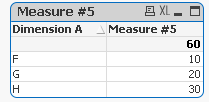QlikView App Dev

Discussion Board for collaboration related to QlikView App Development.

Announcements
QLIKWORLD LIVE! MAY 16 - 19TH, EARLY BIRD DISCOUNTS! REGISTER TODAY
cancel
Showing results for
Did you mean:Not applicable

Set analysis - data island

Hi,

I've come across a tricky set analysis operation.

I have two tables (refer to sample app for further details):

t_fact:

Dimension A, Measure #1, Measure #2

F, 6, 1

F, 5, 2

G, 4, 3

G, 3, 4

H, 2, 5

H, 1, 6

];

t_dataisland:

Measure #3, Measure #4, Measure #5

11, 3, 10

7, 7, 20

3, 11, 30

];

I want to create a simple straight table with Dimension A as Dim and a single expression which should be the sum of [Measure #5). Given that there is no connection between tables I want to use Set Analysis in a way similar to this:

= Sum({<[Measure #3]={"\$(=Sum([Measure #1]))"}, [Measure #4]={"\$(=Sum([Measure #2]))"}>}[Measure #5])

In fact, what I'm saying is that given the sum of Measures #1 and #2, retrieve the value of Measure #5:

Dimension AMeasure #5
F10
G20
H30

I am missing something because I can't get it to work... maybe you'll have better clues than I do.

1 Solution

Accepted SolutionsMVP

Although won't give you an amazing performance, but this expression works:

=Sum(Aggr(If([Measure #3] = Sum([Measure #1]) and [Measure #4] = Sum([Measure #2]), [Measure #5]), [Dimension A], [Measure #3], [Measure #4], [Measure #5]))2 RepliesMVP

Although won't give you an amazing performance, but this expression works:

=Sum(Aggr(If([Measure #3] = Sum([Measure #1]) and [Measure #4] = Sum([Measure #2]), [Measure #5]), [Dimension A], [Measure #3], [Measure #4], [Measure #5]))Not applicable
Author

It certainly works.

I am amazed that this can't be achieved with set analysis.